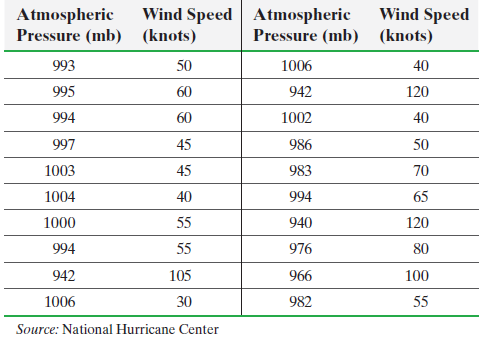×
Get Full Access to Statistics: Informed Decisions Using Data - 5 Edition - Chapter 4.1 - Problem 30
Get Full Access to Statistics: Informed Decisions Using Data - 5 Edition - Chapter 4.1 - Problem 30

×

# ?Hurricanes The data in the next column represent the maximum wind speed (in knots) and atmospheric pressure (in millibars) for a random sample of hurrISBN: 9780134133539 240

## Solution for problem 30 Chapter 4.1

Statistics: Informed Decisions Using Data | 5th Edition

• Textbook Solutions
• 2901 Step-by-step solutions solved by professors and subject experts
• Get 24/7 help from StudySoup virtual teaching assistantsStatistics: Informed Decisions Using Data | 5th Edition

4 5 1 292 Reviews
22
0
Problem 30

Hurricanes The data in the next column represent the maximum wind speed (in knots) and atmospheric pressure (in millibars) for a random sample of hurricanes that originated in the Atlantic Ocean.(a) Draw a scatter diagram treating atmospheric pressure as the explanatory variable.

(b) Compute the linear correlation coefficient between atmospheric pressure and wind speed.

(c) Does a linear relation exist between atmospheric pressure and wind speed?

Step-by-Step Solution:

Step 1 of 5) Hurricanes The data in the next column represent the maximum wind speed (in knots) and atmospheric pressure (in millibars) for a random sample of hurricanes that originated in the Atlantic Ocean. (a) Draw a scatter diagram treating atmospheric pressure as the explanatory variable. (b) Compute the linear correlation coefficient between atmospheric pressure and wind speed. (c) Do linear relationships exist between atmospheric pressure and wind speed Approach Step 1 Plot points for each year, with the date on the horizontal axis and the Partisan Conflict Index on the vertical axis.

Step 2 of 2

## Discover and learn what students are asking

Calculus: Early Transcendental Functions : Differential Equations: Growth and Decay
?In Exercises 1-10, solve the differential equation. $$\frac{d y}{d x}=5-8 x$$

Calculus: Early Transcendental Functions : Iterated Integrals and Area in the Plane
?In Exercises 1 - 10, evaluate the integral. $$\int_{x^{3}}^{\sqrt{x}}\left(x^{2}+3 y^{2}\right) d y$$

Unlock Textbook Solution